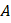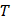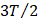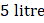# The system shown in the figure is in equilibrium, whereandare isomeric liquids and form an ideal solution atK. Standard vapour pressures ofandareand, respectively, atK. We collect the vapour ofandin two containers of volume, first container is maintained at 2K and second container is maintained at. At the temperature greater thanK, bothandexist in only gaseous form We assume than collected gases behave ideally at 2K and there may take place an isomerization reaction in whichgets converted intoby first-order kinetics reaction given as:whereis a rate constant In container (II) at the given temperature,andare ideal in nature and non reacting in nature. A small pin hole is made into container. We can determine the initial rate of effusion of both gases in vacuum by the expressionWhere,pressure difference between system and surroundingpositive constantmolecular weight of the gas If partial vapour pressure ofis twice that of partial vapour pressure ofand total pressure 2 atm atK, whereK andL, then the number of moles ofandin vapour phase is: a)b)c)d)## Question ID - 103090 :- The system shown in the figure is in equilibrium, whereandare isomeric liquids and form an ideal solution atK. Standard vapour pressures ofandareand, respectively, atK. We collect the vapour ofandin two containers of volume, first container is maintained at 2K and second container is maintained at. At the temperature greater thanK, bothandexist in only gaseous form We assume than collected gases behave ideally at 2K and there may take place an isomerization reaction in whichgets converted intoby first-order kinetics reaction given as:whereis a rate constant In container (II) at the given temperature,andare ideal in nature and non reacting in nature. A small pin hole is made into container. We can determine the initial rate of effusion of both gases in vacuum by the expressionWhere,pressure difference between system and surroundingpositive constantmolecular weight of the gas If partial vapour pressure ofis twice that of partial vapour pressure ofand total pressure 2 atm atK, whereK andL, then the number of moles ofandin vapour phase is: a)b)c)d)3537

(a)Next Question :of gasis placed in a container ofvolume at pressureand temperatureThe gas is heated fromtoIt shows positive deviation,at high pressure.

 Which of the following statement is wrong about the gas? a) The gas is monoatomic b) The gas isc) The gas is a rare gas or inert gas d) The number of molecules of gas in